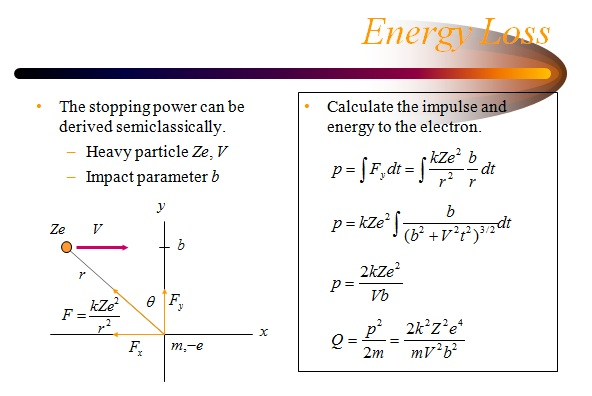## Energy transferDerivation
Kinetic energy of a free particle E= ½mv2 where m is the mass of the electron and v is its velocity.
Momentum p=mv so v=p/m
Substituting for v we get E=½mp2/m2, or

E=p2/2m

k=1/4πε0